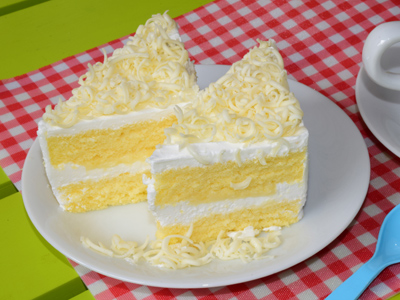What would you rather have: a half of a half of a half of something nice or a half of a quarter of something nice?

# Fractions (Medium)

Fractions have a denominator and a numerator. In your lessons at school you will no doubt be learning all about fractions. They can sometimes cause confusion and if you haven't quite understood them so far, keep playing our quizzes until you are sure you understand what they mean.

You may think fractions are old-fashioned and no longer used, but you will come across them in everyday life often enough. To test this, while you are out and about this week, see how many fractions you can spot - and more importantly, see if you can work out what they mean!

In this 11-plus Maths quiz you will get a chance to revise what you have already learnt. Do the easier quizzes on fractions BEFORE you do this quiz.

Take your time and try and have fun.

1.
How many sevenths are there in 6?
35
49
21
42
There are seven sevenths in 1, so there are 7 × 6 = 42 sevenths in 6. In general, the number in the denominator tells you how many fractions of that type are needed to make 1. For example, you need three thirds, 13, to make 1, but you need ten tenths, 110, to make 1 and so on. REMEMBER this stuff, and you won't get confused with fractions
2.
What would you rather have: a half of a half of a half of something nice or a half of a quarter of something nice?
A half of a quarter
They are both the same, so it doesn't matter which one you pick
A half of a half of a half
A half of a half
12 × 12 × 12 = 14 × 12 = 18. The word OF means multiply
3.
What is 125 as a mixed fraction?
2212
1225
225
525
To convert an improper fraction to a mixed fraction, follow these steps: 1. Divide the numerator by the denominator. 2. Note the whole number remainder. 3. Write the number from step 1 as the whole number in front of the fractional part AND write the fractional part with the remainder in the numerator and keep the original denominator. STEP 1: 12 ÷ 5 = 2. STEP 2: Remainder 2. STEP 3: 225
4.
What is 123 as a mixed fraction?
123
1213
4
12
To convert an improper fraction to a mixed fraction, follow these steps: 1. Divide the numerator by the denominator. 2. Note the whole number remainder. 3. Write the number from step 1 as the whole number in front of the fractional part AND write the fractional part with the remainder in the numerator and keep the original denominator. STEP 1: 12 ÷ 3 = 4. STEP 2: Remainder 0. STEP 3: 4. Be on the LOOKOUT for this sort of thing!
5.
What is 435 as an improper fraction?
205
125
75
235
To convert a mixed fraction to an improper fraction, follow these steps: 1. Multiply the whole number part by the denominator. 2. Add this result to the numerator. 3. Write the fraction with step 2 in the numerator and keep the original denominator. STEP 1: 4 × 5 = 20. STEP 2: 20 + 3 = 23. STEP 3: 235
6.
What is 98 - 34 + 58
1
118
118
208
98 - 34 + 58 = 98 - 68 + 58 = 1. Reduce the fractions to their simplest forms, then add them. If the denominators are the same, you can add the fractions by simply adding their numerators - it really is as easy as that!
7.
What is 25 + 410 + 1215?
1615
263
85
1810
25 + 410 + 1215 = 25 + 25 + 45 = 85. Reduce the fractions to their simplest forms, then add them. If the denominators are the same, you can add/subtract the fractions by simply adding/subtracting their numerators - it really is as easy as that!
8.
What is two-thirds of £81?
£18
£54
£64
£27
23 × £81 = (2 × £81) ÷ 3 = £162 ÷ 3 = £54. Do the brackets first. The word OF means multiply
9.
What is 823 as an improper fraction?
243
163
263
103
To convert a mixed fraction to an improper fraction, follow these steps: 1. Multiply the whole number part by the denominator. 2. Add this result to the numerator. 3. Write the fraction with step 2 in the numerator and keep the original denominator. STEP 1: 8 × 3 = 24. STEP 2: 24 + 2 = 26. STEP 3: 263
10.
What is 23 of 21?
7
14
18
12
23 × 21 = (2 × 21) ÷ 3 = 42 ÷ 3 = 14. Do the brackets first. The word OF means multiply
Author:  Frank Evans